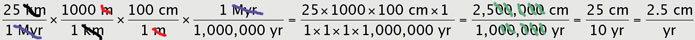## Unit Conversions can also be called:

Dimensional analysis
Unit analysis
Factor label method

# How do I change units on a number? Unit conversions in the geosciences

## Introduction to unit conversions

In the geosciences, we think about how the Earth works on a variety of scales. For example, the San Andreas Fault that runs nearly the length of California's coastline is over 1200 km long. But we talk about movement on the fault in terms of mm per year. So, we have to be able to think about thousands of km and a few mm when talking about the same feature. In addition, a geoscientist who lives in the United States needs to be able to think in terms of English and metric units - the public thinks in terms of English units (miles, gallon, etc.) and the scientific community uses SI units (kilometers, liters, etc.). This may seem difficult at first, but it is crucial for anyone studying geosciences to be able to move easily from one unit to another (with a little calculation, of course). Luckily, there are some simple steps that, if followed, can help you complete unit conversions with relative ease.

## How do I do a unit conversion?

You can do any unit conversion if you follow a few simple steps. Although there is no single "right" way to do unit conversions, these steps provide one way to learn to do unit conversions. DO NOT SKIP ANY STEPS! Although it may seem tedious, working through unit conversions requires that each of these steps be followed so that you can be sure that you end up with what you want, especially when you are just starting out with learning to do unit conversions.

• If you are converting from one metric unit to another, this list of metric prefixes (Acrobat (PDF) 7kB Aug31 11) will be useful.
• When converting from metric to imperial (sometimes called English) units (orvice versa), this conversion chart (Acrobat (PDF) 40kB Sep3 09) might be helpful.

When you do any unit conversion, you should always know what units you started with and what units you want to end up with. This is key to success at unit conversions.

## The Steps

The steps to successfully completing a unit conversion are outlined below. To illustrate the steps, lets use a geologic example: In Southern California, slip on the San Andreas Fault is on the order of 25 km/Myr. How many cm does the San Andreas Fault move in one year?
1. Write (copy) out the units that you are given as a fraction.
2. Write out the units that you want at the end of the conversion as a fraction:
3. Determine appropriate conversion factors. Use tables in your textbook or download one of the tables listed above (for this particular example, you probably only need to know the prefixes for metric system (Acrobat (PDF) 7kB Aug31 11)).
4. Evaluate the appropriate arrangement for conversion factors. That is, copy the conversion factor(s) from step 3 into fraction form so that the units end up canceling. Remember that when you multiply fractions (as you will in step 6 below), you can cancel units ONLY when they appear in the numerator of one fraction and the denominator of another.
5. Set up the conversion by writing the fractions in a row with multiplication signs in between.
6. Evaluate. Do the original units cancel so that you end up with what the question is asking for? If not, repeat steps 2 and 3 until they do!
7. Now, lets do some arithmetic: To complete the conversion, we multiply all the numerators and then all the denominators (or multiply across the top and bottom).
8. Reduce the fraction by dividing numerator by denominator once you have the top and bottom multiplied.
9. EVALUATE (again). Is this a reasonable number?

### You can download and print a sheet with the unit conversion steps(Acrobat (PDF) 44kB Apr11 08)

to use while completing the practice problems.

Just a quick note about the metric system: Although the US is one of the last hold outs on the imperial or English system of measurement (inches, miles, °F, etc.), the metric system is often much more intuitive because it is a system of base 10 numbers. The nice thing about dividing numbers in the metric system is that when you get to the end, you can actually cancel out the zeros in the fraction (remember, you have to cancel the same number on the top and bottom - there may be a few left over in either the numerator or the denominator). For example in the question above:so that you only have to divide 25 by 10. If you are rusty about what the prefixes mean for the metric system, you can review the order of magnitude for a "femtogram" or "gigabyte" or any other SI unit using the metric prefixes (Acrobat (PDF) 7kB Aug31 11) document available earlier on the page.

## Where are unit conversions used in the geosciences?

• Plate tectonics - converting rates of plate motion, etc.
• Topographic maps - converting scales
• Rivers and Streams - converting rates of flow, slope, etc.
• Groundwater - converting rates of flow
• Glaciers - converting rates of flow (or retreat), etc.
• Geologic time - converting time, rates of deposition, etc.
And many other topics...